Courses

# Test: Difference Amplifiers

## 10 Questions MCQ Test Electronic Devices | Test: Difference Amplifiers

Description
This mock test of Test: Difference Amplifiers for Electrical Engineering (EE) helps you for every Electrical Engineering (EE) entrance exam. This contains 10 Multiple Choice Questions for Electrical Engineering (EE) Test: Difference Amplifiers (mcq) to study with solutions a complete question bank. The solved questions answers in this Test: Difference Amplifiers quiz give you a good mix of easy questions and tough questions. Electrical Engineering (EE) students definitely take this Test: Difference Amplifiers exercise for a better result in the exam. You can find other Test: Difference Amplifiers extra questions, long questions & short questions for Electrical Engineering (EE) on EduRev as well by searching above.
QUESTION: 1

### For the difference amplifier which of the following is true?

Solution:

All the statements are not true except for the fact that it responds only when there is difference between two signals only.

QUESTION: 2

### If for an amplifier the common mode input signal is vc, the differential signal id vd and Ac and Ad represent common mode gain and differential gain respectively, then the output voltage v0is given by

Solution:

It is a standard mathematical expression.

QUESTION: 3

### . If for an amplifier v1 and v2 are the input signals, vc and vd represent the common mode and differential signals respectively, then the expression for CMRR (Common Mode Rejection Ratio) is

Solution:

Note that all the expressions are identical.

QUESTION: 4

The problem with the single operational difference amplifier is its

Solution:

Due to low input resistance a large part of the signal is lost to the source’s internal resistance.

QUESTION: 5

For the difference amplifier as shown in the figure show that if each resistor has a tolerance of ±100 ε % (i.e., for, say, a 5% resistor, ε = 0.05) then the worst-case CMRR is given approximately by (given K = R2/R1 = R4/R3)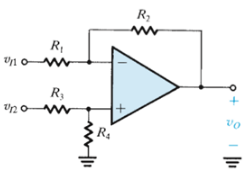Solution:
QUESTION: 6

For the circuit given below determine the input common mode resistance.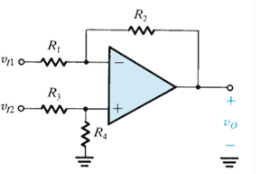Solution:

Parallel combination of series combination of R1 & R3 with the series combination of R3 and R4 is the required answer as is visible by the circuit.

QUESTION: 7

For the circuit shown below express v0 as a function of v1 and v2.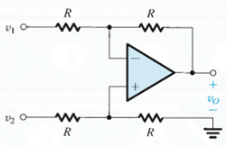Solution:

Considering the fact that the potential at the input terminals are identical and proceeding we obtain the given result.

QUESTION: 8

For the difference amplifier shown below, let all the resistors be 10kΩ ± x%. The expression for the worst-case common-mode gain is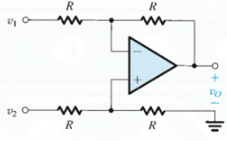Solution:
QUESTION: 9

Determine Ad and Ac for the given circuit.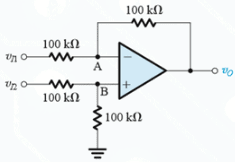Solution:

Consider the fact that the potential at the input terminals are identical and obtain the values of V1 and V2. Thus obtain the value of Vd and Vc.

QUESTION: 10

Determine the voltage gain for the given circuit known that R1 = R3 = 10kΩ abd R2 = R4 = 100kΩ.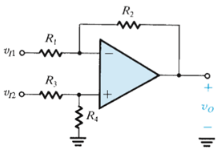Solution:

Voltage gain is 100/10.